# Combinations

From how many elements we can create 990 combinations 2nd class without repeating?

n =  45

### Step-by-step explanation: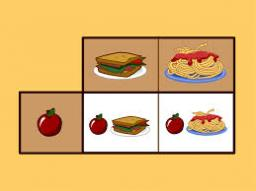Did you find an error or inaccuracy? Feel free to write us. Thank you!Tips to related online calculators
Looking for help with calculating roots of a quadratic equation?
Would you like to compute count of combinations?

## Related math problems and questions:

• 2nd class combinationsFrom how many elements you can create 4560 combinations of the second class?
• CombinationsIf the number of elements increase by 3, it increases the number of combinations of the second class of these elements 5 times. How many are the elements?
• CombinationsHow many elements can form six times more combinations fourth class than combination of the second class?
• Variations 3rd classFrom how many elements we can create 13,800 variations 3rd class without repeating?
• 2nd class variationsFrom how many elements you can create 2450 variations of the second class?
• Variations 4/2Determine the number of items when the count of variations of fourth class without repeating is 600 times larger than the count of variations of second class without repetition.
• Combinations 66 purses 9 flaps 12 straps Every combination must include 1 purse, 1 flap, and 1 strap. How many are possible combinations?
• Elements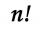If the number of elements is decreased by two the number of permutations is decreased 30 times. How many elements are?
• Two-element combinations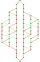Write all two-element combinations from elements a, b, c, d.
• VCP equationSolve the following equation with variations, combinations and permutations: 4 V(2,x)-3 C(2,x+ 1) - x P(2) = 0
• Class pairs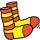In a class of 34 students, including 14 boys and 20 girls. How many couples (heterosexual, boy-girl) we can create? By what formula?
• MartinaMartina is solving the equation 4x - 11 = 2x + 391. Here are the first steps of her solution. 4x - 11 = 2x + 391 2x - 11 = 391 2x = 402 What did Martina do to get 2x - 11 = 391?
• Statistics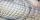The sum of all deviations from the arithmetic mean of the numerical sequence 4, 6, 51, 77, 90, 93, 95, 109, 113, 117 is:
• Family94 boys are born per 100 girls. Determine the probability that there are two boys in a randomly selected family with three children.
• I think numberI think number.When I add 841 to it and subtract 157, I get a number that is 22 greater than 996. What number I thinking?
• A photographA photograph will stick to a white square letter with a x cm length. The photo is 3/4 x cm long and 20 cm wide than the width of the paper. The surface of the remaining paper surrounding the photograph is 990 cm2. Find the size of paper and photo.
• Party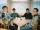At the party everyone clink with everyone. Together, they clink 406 times. How many people were at the party?